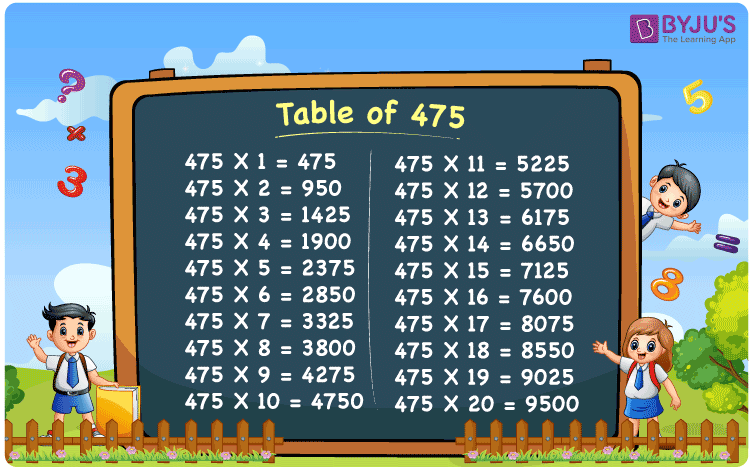Checkout JEE MAINS 2022 Question Paper Analysis : Checkout JEE MAINS 2022 Question Paper Analysis :

# Table of 475

The table of 475, or the 475 times table, is the multiplication table that results in the multiplication of the number 475 with consecutive natural numbers. Learning the multiplication table of 475 will assist students in saving a significant amount of time during the examination. Also, the 475 times table is provided in the PDF so that students can easily download the table of 475 and learn offline.

## Table of 475 Chart## What is the 475 Times Table?

The 475 times table can be written using repeated addition of 475, along with the multiplication of 475 by natural numbers. The below table shows the results of the 475 times table up to 10 times.

 Using multiplication Using repeated addition 475 × 1 = 475 475 475 × 2 = 950 475 + 475 = 950 475 × 3 = 1425 475 + 475 + 475 = 1425 475 × 4 = 1900 475 + 475 + 475 + 475 = 1900 475 × 5 = 2375 475 + 475 + 475 + 475 + 475 = 2375 475 × 6 = 2850 475 + 475 + 475 + 475 + 475 + 475 = 2850 475 × 7 = 3325 475 + 475 + 475 + 475 + 475 + 475 + 475 = 3325 475 × 8 = 3800 475 + 475 + 475 + 475 + 475 + 475 + 475 + 475 = 3800 475 × 9 = 4275 475 + 475 + 475 + 475 + 475 + 475 + 475 + 475 + 475 = 4275 475 × 10 = 4750 475 + 475 + 475 + 475 + 475 + 475 + 475 + 475 + 475 + 475 = 4750

## Multiplication Table of 475

Go through the multiplication table of 475 given below to learn the first 20 multiples of 475.

 475 × 1 = 475 475 × 2 = 950 475 × 3 = 1425 475 × 4 = 1900 475 × 5 = 2375 475 × 6 = 2850 475 × 7 = 3325 475 × 8 = 3800 475 × 9 = 4275 475 × 10 = 4750 475 × 11 = 5225 475 × 12 = 5700 475 × 13 = 6175 475 × 14 = 6650 475 × 15 = 7125 475 × 16 = 7600 475 × 17 = 8075 475 × 18 = 8550 475 × 19 = 9025 475 × 20 = 9500

## Solved Example

Question:

Using the table of 475, calculate: 475 times 17 + 2200

Solution:

475 times 17 + 2200

= 475 × 17 + 2200

Using the table of 475, 475 × 17 = 8075.

= 8075 + 2200

= 10275

Therefore, the value of 475 times 17 + 2200 is 10275.

## Frequently Asked Questions on Table of 475

### What is the multiplication table of 475?

The multiplication table of 475 denotes the multiplication of 475 by numbers 1, 2, 3, and so on. We can also create the multiplication table of 475 using the repeated addition of 475.

### Write the table of 475 up to 10 times.

The table of 475 up to 10 times is given as: 475 × 1 = 475, 475 × 2 = 950, 475 × 3 = 1425, 475 × 4 = 1900, 475 × 5 = 2375, 475 × 6 = 2850, 475 × 7 = 3325, 475 × 8 = 3800, 475 × 9 = 4275, 475 × 10 = 4750

### How many times should we add 475 to get 2850?

We should add 475 for six times to get 2850. As we know, 475 × 6 = 2850 so 475 + 475 + 475 + 475 + 475 + 475 = 2850.